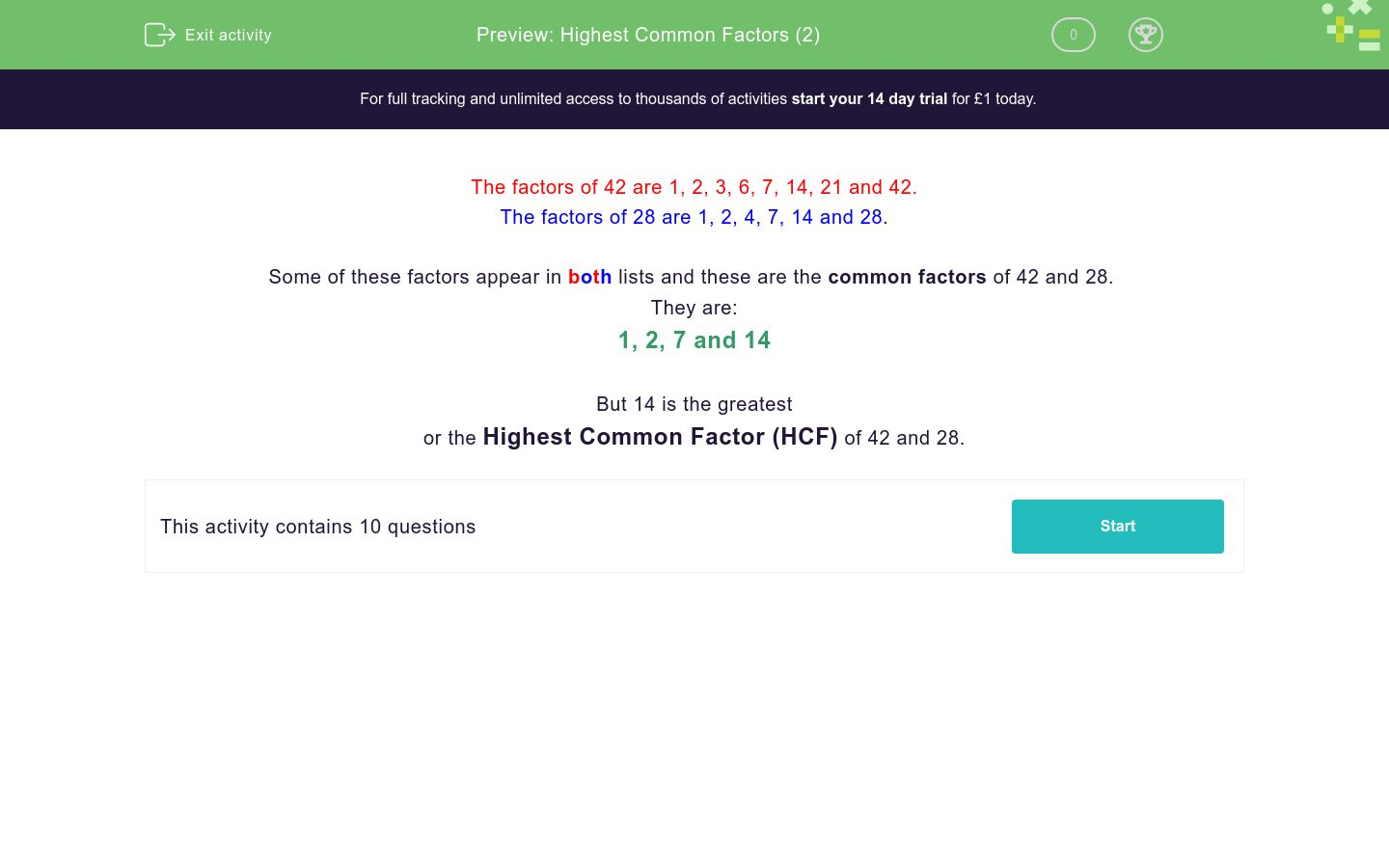# Highest Common Factors (2)

In this worksheet, students find the highest common factor of two numbers.Key stage:  KS 3

Curriculum topic:   Number

Curriculum subtopic:   Use Concepts and Vocabulary for All Numbers

Difficulty level:### QUESTION 1 of 10

The factors of 42 are 1, 2, 3, 6, 7, 14, 21 and 42.

The factors of 28 are 1, 2, 4, 7, 14 and 28.

Some of these factors appear in both lists and these are the common factors of 42 and 28.

They are:

1, 2, 7 and 14

But 14 is the greatest

or the Highest Common Factor (HCF) of 42 and 28.

What is the highest common factor of....?

42 and 12

What is the highest common factor of....?

12 and 36

What is the highest common factor of....?

30 and 50

What is the highest common factor of....?

30 and 60

What is the highest common factor of....?

30 and 45

What is the highest common factor of....?

16 and 24

What is the highest common factor of....?

40 and 56

What is the highest common factor of....?

42 and 56

What is the highest common factor of....?

42 and 72

What is the highest common factor of....?

42 and 105

• Question 1

What is the highest common factor of....?

42 and 12

6
EDDIE SAYS
The first step here is to find all the factors of the numbers; Factors of 42 = 1, 2, 3, 6, 7, 14. 21, 42 Factors of 12 = 1, 2, 3, 4, 6, 12 The highest common factor (HCF) is the largest number that is in both lists
• Question 2

What is the highest common factor of....?

12 and 36

12
EDDIE SAYS
The first step here is to find all the factors of the numbers; Factors of 12 = 1, 2, 3, 4, 6, 12 Factors of 36 = 1, 2, 3, 4, 6, 9, 12, 18, 36 The highest common factor (HCF) is the largest number that is in both lists
• Question 3

What is the highest common factor of....?

30 and 50

10
EDDIE SAYS
The first step here is to find all the factors of the numbers; Factors of 30 = 1, 2, 3, 5, 6, 10, 15, 30 Factors of 50 = 1, 2, 5, 10, 25, 50 The highest common factor (HCF) is the largest number that is in both lists
• Question 4

What is the highest common factor of....?

30 and 60

30
EDDIE SAYS
The first step here is to find all the factors of the numbers; Factors of 30 = 1, 2, 3, 5, 6, 10, 15, 30 Factors of 60 = 1, 2, 3, 4, 5, 6, 10, 12, 15, 20, 30, 60 The highest common factor (HCF) is the largest number that is in both lists
• Question 5

What is the highest common factor of....?

30 and 45

15
EDDIE SAYS
The first step here is to find all the factors of the numbers; Factors of 30 = 1, 2, 3, 5, 6, 10, 15, 30 Factors of 45 = 1, 3, 5, 9, 15, 45 The highest common factor (HCF) is the largest number that is in both lists
• Question 6

What is the highest common factor of....?

16 and 24

8
EDDIE SAYS
The first step here is to find all the factors of the numbers; Factors of 16 = 1, 2, 4, 8, 16 Factors of 32 = 1, 2, 4, 8, 16, 32 The highest common factor (HCF) is the largest number that is in both lists
• Question 7

What is the highest common factor of....?

40 and 56

8
EDDIE SAYS
The first step here is to find all the factors of the numbers; Factors of 40 = 1, 2, 4, 5, 8, 10, 20, 40 Factors of 56 = 1, 2, 4, 7, 8, 14, 28, 56 The highest common factor (HCF) is the largest number that is in both lists
• Question 8

What is the highest common factor of....?

42 and 56

14
EDDIE SAYS
The first step here is to find all the factors of the numbers; Factors of 42 = 1, 2, 3, 6, 7, 14, 21, 42 Factors of 56 = 1, 2, 4, 7, 8, 14, 28, 56 The highest common factor (HCF) is the largest number that is in both lists
• Question 9

What is the highest common factor of....?

42 and 72

6
EDDIE SAYS
The first step here is to find all the factors of the numbers; Factors of 42 = 1, 2, 3, 6, 7, 14, 21,42 Factors of 72 = 1, 2, 3, 4, 6, 8, 9, 12, 18, 36, 72 The highest common factor (HCF) is the largest number that is in both lists
• Question 10

What is the highest common factor of....?

42 and 105

21
EDDIE SAYS
The first step here is to find all the factors of the numbers; Factors of 42 = 1, 2, 3, 6, 7, 14, 21, 42 Factors of 105 = 1, 3, 5, 7, 15, 21, 35, 105 The highest common factor (HCF) is the largest number that is in both lists
---- OR ----

Sign up for a £1 trial so you can track and measure your child's progress on this activity.

### What is EdPlace?

We're your National Curriculum aligned online education content provider helping each child succeed in English, maths and science from year 1 to GCSE. With an EdPlace account you’ll be able to track and measure progress, helping each child achieve their best. We build confidence and attainment by personalising each child’s learning at a level that suits them.

Get started